﻿ Sector Weighting: A Detailed Implementation of Black-LittermanFull R-Markdown Source code for this document can be downloaded here along with sector returns .csv here.

This report will provide a detailed walkthrough in arriving at an optimal sector allocation in line to our Equity managers’ beliefs of relative returns between sectors. Optimal sector allocation is derived using a well-known model called “Black-Litterman”, which I will refer to as BL henceforth. BL is implemented as an improvement over the traditional Markowitz portfolio optimization through

1. incorporating investor beliefs about returns on asset through Bayesian inference
2. solving the problem of overly concentrated portfolios with a process called reverse optimization.

Lastly, final sector weighting will be subjectively adjusted from the model output to ensure mandates are accounted for e.g maximum 25% allocation for a sector.

In this report, detailed implementation of BL is shown along with supporting r code to clarify process. The report is suppose to be intuitive and goal-oriented. Auxiliary equations or derivations are beyond the scope of this report. Methodology is precisely followed from Thomas Idzorek (2005).

### Defining Sector Returns

SSIF mandate defines its benchmark to be 65% US equity, 30% Canadian equity and 5% 10-yr CAD Treasury. Since treasury rates do not relate to sectors, we will assume 65% US and 35% CN Allocation. There are ten sectors from both countries and we combine these returns into a single sector according to these allocations.

Five year monthly returns are obtained from Bloomberg indices and the closest proxies I use are the S&P 500 (US) and S&P/TSX (CA) Indices.

  head(r,n=2) #illustrate sector returns csv files
##         Date US.CONS.DISCRET US.CONS.STAPLES   US.ENERGY US.HEALTH.CARE
## 1 31/05/2010     -0.07142427     -0.04734875 -0.11807642    -0.06992070
## 2 30/06/2010     -0.09802833     -0.02801525 -0.05790921    -0.01861415
##   US.FINANCIALS US.INFO.TECH US.UTILITIES US.INDUSTRIALS US.MATERIALS
## 1   -0.09248874  -0.08273147  -0.06121656    -0.09826671  -0.09651044
## 2   -0.05966974  -0.06216600  -0.01021253    -0.07092171  -0.07099763
##   US.TELECOM.SERV CN.CONS.DIS  CN.CONS.STP   CN.ENERGY  CN.HLTH.CR
## 1    -0.039384846  0.01261650 -0.003101773 -0.04642476 -0.02415584
## 2    -0.003904725 -0.02345942 -0.039391805 -0.04966424  0.07505989
##     CN.FINCLS CN.INFO.TECH     CN.UTIL CN.INDSTRLS   CN.MATRLS CN.TEL.SRVC
## 1 -0.05488357  0.004033613 -0.06121656 -0.09826671 -0.09651044  0.00071908
## 2 -0.05694311 -0.089052561 -0.01021253 -0.07092171 -0.07099763 -0.00502994

We now combine these returns into a single sector and plot it to ensure everything looks good. Plotted are cumulative compounded returns from each combined sectors.

  r.us <- r[,2:11] # US Sector Returns
r.cn <- r[,-(1:11)] # CN Sector Returns
r.comb <- 0.65*r.us + 0.35*r.cn #Combine them according to allocation

rownames(r.comb) <- r[,1]
colnames(r.comb) <- c("CONS.DISCRET", "CONS.STAPLES", "ENERGY", "HEALTH.CARE", "FINANCIALS", "INFO.TECH", "UTILITIES", "INDUSTRIALS", "MATERIALS", "TELECOM")

matplot(cumprod(1+r.comb), type = c("l"), col=1:10, ylab="Cumulative Return", xlab="T") #plot cumulative compounded returns
legend("topleft", legend = colnames(r.comb), col=1:10,pch=1,cex=0.6) # legend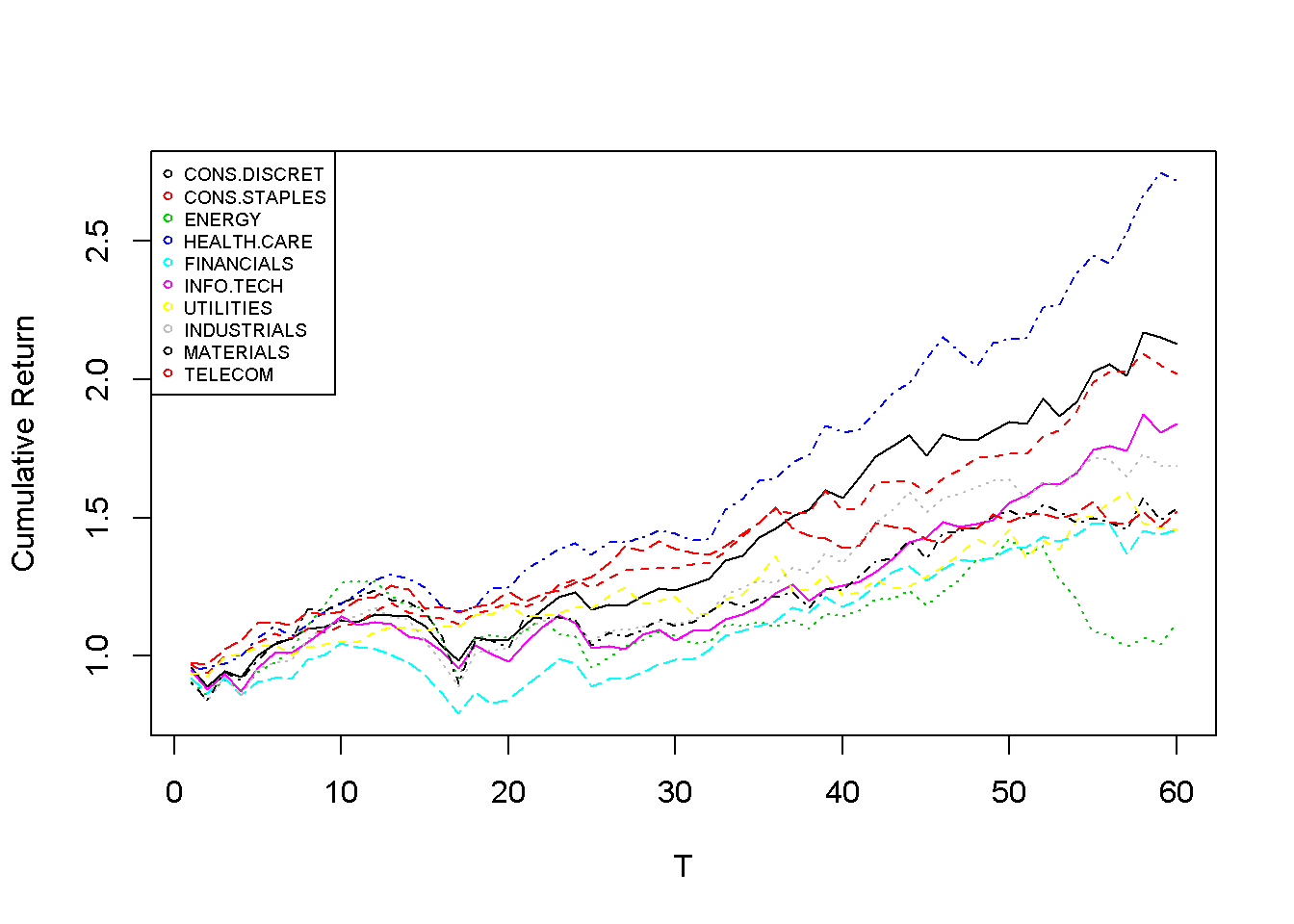Monthly sector returns are used to estimate the covariance matrix $$\Sigma$$. Due to estimation risk prevalent in asset returns, I will use the constant correlation model to estimate the covariance matrix.

  sigma <- apply(r.comb,2,sd) #Get Standard deviation of sectors
rho <- cor(r.comb) # Get Correlation matrix
cc <- mean(upper.tri(rho, diag=FALSE)) # Get Average pairwise correlation
rho[rho != 1] <- cc
cov.mat <- rho * (sigma %*% t(sigma))

### Reverse Optimization

In this section, I estimate the implied optimal returns for BL. This process is done through reverse optimization by first assuming that the market capitalization weights $$w_{mkt}$$ are considered “optimal” and then deriving implied returns through the covariance matrix. More specifically, implied returns are scaled from $$(\Sigma) w_{mkt}$$ with $$\lambda$$, often referred to as the risk aversion coefficient. Lambda can be estimated as such:

$\lambda = \frac{E[R]-r_f}{\sigma^2}$

  # Both US and CN sector returns are used to calculate lambda since combined weightings do not provide accurate actual historical return.
# Weightings as of end of april 2015
w.mkt.us <- c(0.125,0.095,0.085,0.146,0.161,0.199,0.03,0.103,0.032,0.023)
w.mkt.cn <- c(0.062,0.035,0.222,0.053,0.347,0.024,0.022,0.081,0.109,0.046)

# Get Annual Expected Return
ret.total <- as.matrix(r.us)%*%(0.65*w.mkt.us)+as.matrix(r.cn)%*%(0.35*w.mkt.cn)
er <- mean(ret.total)*12
er
##  0.1089661
  rf <- 0.02 # 10 Y Annual US Yield are about 2%
v <- var(ret.total) # Variance of the total return
lambda <- (er-rf)/v
lambda
##          [,1]
## [1,] 75.78372

We can now calculate the implied equilibrium returns using reverse optimization ($$\pi=\lambda\Sigma w_{mkt}$$). We set the market cap weights ($$w_{mkt}$$) to equal to 65% US Weight + 35% CN Weights, same as the benchmark. The reason this works and not in the lambda case is because we are assuming that the combination of the two weights is already “optimal” and deriving the implied expected returns whereas calculating lambda requires accurate historical returns.

  w.mkt <- (0.65*w.mkt.us+0.35*w.mkt.cn)
pi <- c(lambda)*(cov.mat%*%w.mkt)
barplot(t(pi*100), cex.names=0.5, las=2, main="Sector Implied Returns in Percentages")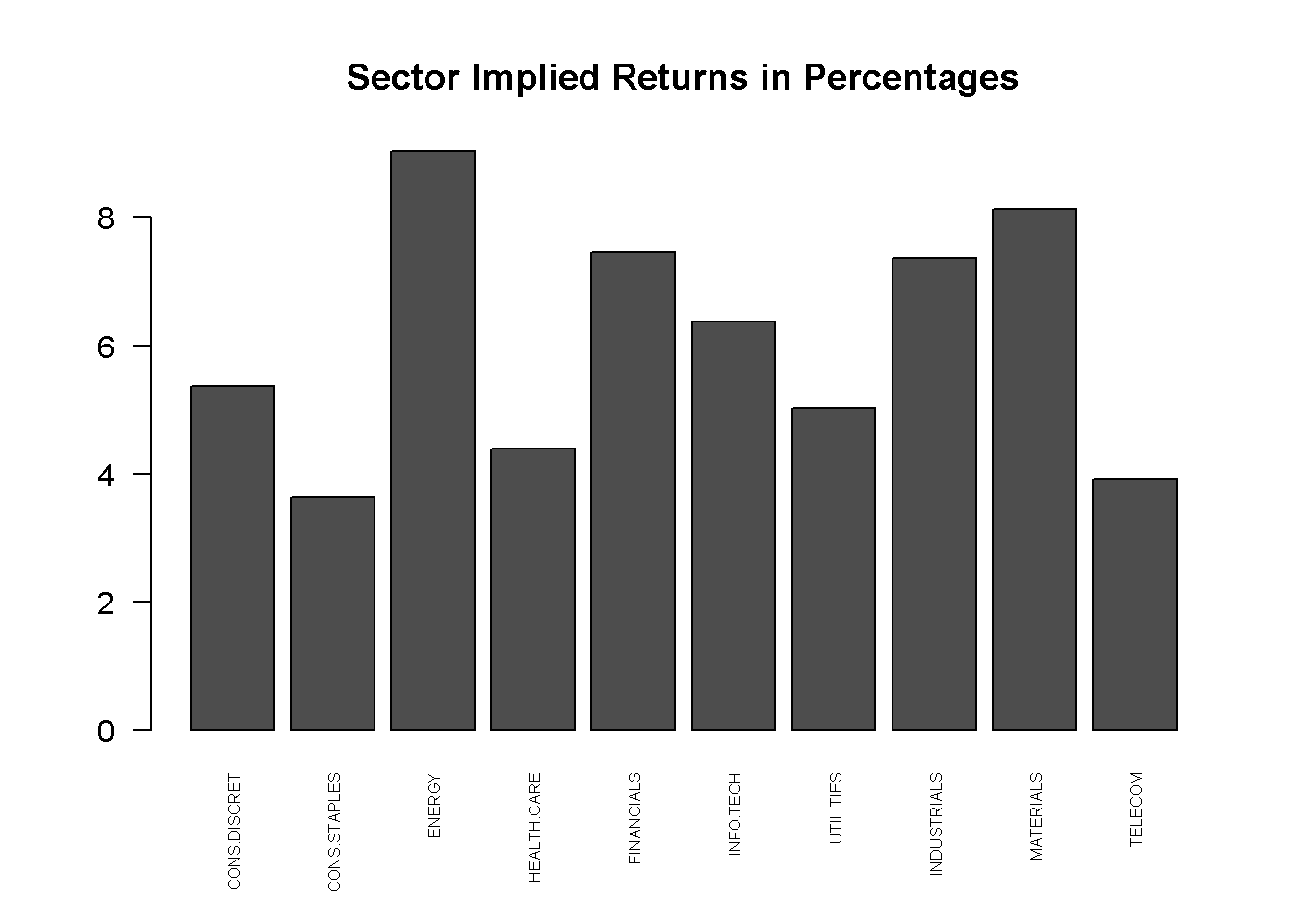### Manager’s Beliefs

In this section, I incorporate our equity manager’s belief system through translating an over/under-weight table into more structured beliefs used in BL. Below is a list of our equity managers’ views on sector positioning relative to current benchmark.

• Consumer Discretionary: Equal Weight with 80% Confidence
• Consumer Staples: Equal Weight with 70% Confidence
• Energy: Equal Weight moving towards Overweight over time with 70% Confidence
• Healthcare: Overweight with 80% Confidence
• Financials: Underweight
• Information Tech.: Overweight with 60% Confidence
• Utilities: Overweight with 80% Confidence
• Industrials: Equal weight with 90% Confidence
• Materials: Underweight
• Telecom: Underweight

#### BL Belief Structure

I am going to introduce an assumption in transforming our previously agreed upon beliefs into the BL belief structure. Within BL, beliefs are structure into three parameters: the impact vector ($$Q$$), the effect matrix ($$P$$) and belief error matrix ($$\Omega$$). Intuitively, the BL belief structure requires the magnitudes of beliefs ($$Q$$ and $$P$$) and the degree of uncertainty regarding these beliefs ($$\Omega$$).

Assumption Sector overweights are believed to outperform both equal weight and underweight sectors. Equal weight sectors are believed to outperform only underweight sectors.

For example, if we expect Health care to outperform, the BL belief is stated such that Health care sector will outperform all sectors, except Info Tech and Utilities, equally by an $$X\%$$ expected annual return.

The Impact Vector
$$Q$$ is a vector with $$K$$ number of beliefs that states the impact of the belief. For example, if we had $$K=1$$ belief that financials would have a 6% return this year, then $$Q=[0.06]$$. We have 3 overweight and underweights, thus we will have 6 beliefs plus one energy thesis discussed in the next paragraph. For now, we will specify the impact to be an arbitrary 5% annual return.

An interesting belief to consider is our energy thesis. One way to interpret this is that currently our managers/analysts are bullish on energy but current low oil prices make it hard to know exactly where stock prices are going. Thus, moving from equal weight to overweight implies that there may be potential rallies from the energy sectors that we want to be more positioned toward but higher gains are more promising in the future (presumably beyond current analysis period). To account for this, the view I will add is the same as overweighting energy except with a lower return potential of 3% as well as less outperformance against equal weights.

  Q <- c(0.05,0.05,0.05,0.05,0.05,0.05,0.03)

The Effect Matrix
$$P$$ is a $$K\times N$$ matrix where we specify what sectors are affected from each belief. For example, if we have three sectors A,B, and C, a belief that A will outperform C is equivalent to $$P = [1,0,-1]$$. The sum of each row must equal to zero according to Idzorek.

    # Heavy positioning in financials for beliefs 1-6 are explained in the last section
P <- rbind(
c(-1/9,-1/9,-1/9, 1 ,-3/9,0,0,-1/9,-1/9,-1/9), #Health care overweight
c(-1/9,-1/9,-1/9,0,-3/9, 1 ,0,-1/9,-1/9,-1/9), #Info Tech overweight
c(-1/9,-1/9,-1/9,0,-3/9,0, 1 ,-1/9,-1/9,-1/9), #Utilities overweight
c(1,0,0,0,-3/5,0,0,0,-1/5,-1/5), #CD Equal Weight
c(0,1,0,0,-3/5,0,0,0,-1/5,-1/5), #CS equal weight
c(0,0,0,0,-3/5,0,0,1,-1/5,-1/5), #Industrials Equal Weight
c(-1/9,-1/9, 1 ,0,-2/9,0,0,-1/9,-2/9,-2/9) # Energy Play
) 

The Uncertainty Matrix
$$\Omega$$ is a $$K\times K$$ diagonal-positive matrix specifying the variance of each view. We assume each view is independent of each other and use the method introduced by Idzorek to specify the confidence. For now, they will be set using $$P_i^T \Sigma P_i \quad \forall i...K$$, intuitively, this can be interpreted as the volatility of constructing a portfolio based on the kth belief. I will show in later section that this becomes irrelevant as we calibrate $$\Omega$$ with confidence levels.

  K <- dim(P)
tau <- 0.01 # Irrelevant for now
omega <- matrix(0,K,K) #Declare empty matrix
# Set diagonal fields
for(i in 1:K){
omega[i,i] <- t(P[i,]) %*% (cov.mat %*% P[i,])
}

### BL Weighting & Returns

The expected return from mixing both optimal implied returns and managers’ beliefs is stated bluntly:

$E[R] = [(\tau\Sigma)^{-1}+P^T\Omega^{-1}P]^{-1}[(\tau\Sigma)^{-1}\pi+P^T\Omega^{-1}Q]$

Likewise, for anyone that has taken STAT2607, the return of each asset is said to follow a normal distribution with an expected return of the above equation $$E[R]$$ and a variance of $$[(\tau\Sigma)^{-1}+P^T\Omega^{-1}P]^{-1}$$. Through normal optimization (reverse reverse optimization), we can apply the new sector returns to get weightings.

$w^* = (\lambda\Sigma)^{-1}E[R]$

  bl.er <- solve(solve(tau*cov.mat)+t(P)%*%(solve(omega)%*%P)) %*% (solve(tau*cov.mat)%*%pi+t(P)%*%(solve(omega)%*%Q))
barplot(t(cbind(pi*100,bl.er*100)), beside=TRUE, col=2:3, cex.names=0.5, las=2, main="Sector Returns in Percentages", sub="Red = Old Implied, Green= New BL")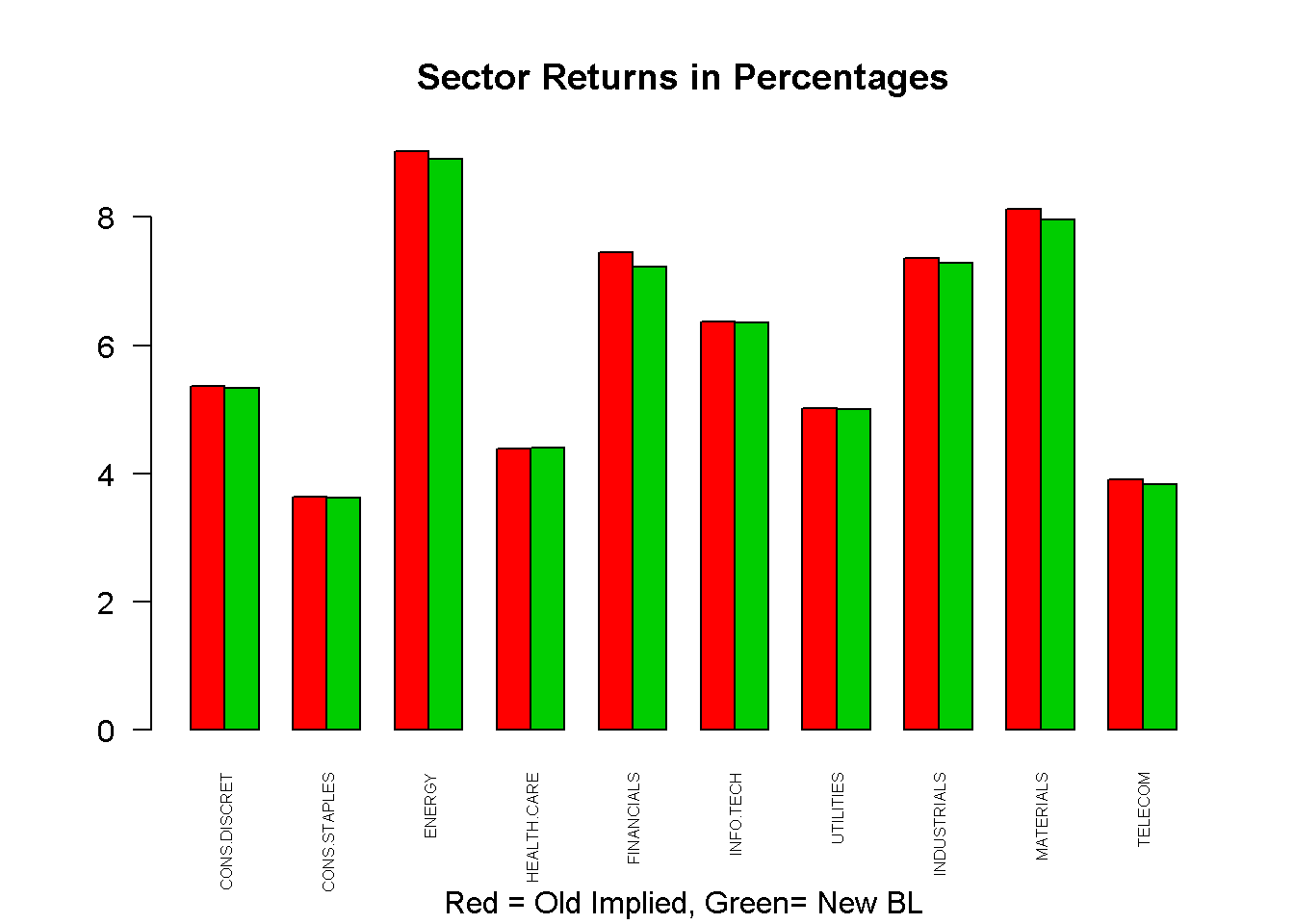w.bl <- solve(c(lambda)*cov.mat)%*%bl.er
barplot(t(cbind(w.mkt*100,w.bl*100)), beside=TRUE, col=2:3, cex.names=0.5, las=2, main="Sector Weighting in Percentages", sub="Red = Market Cap, Green= New BL")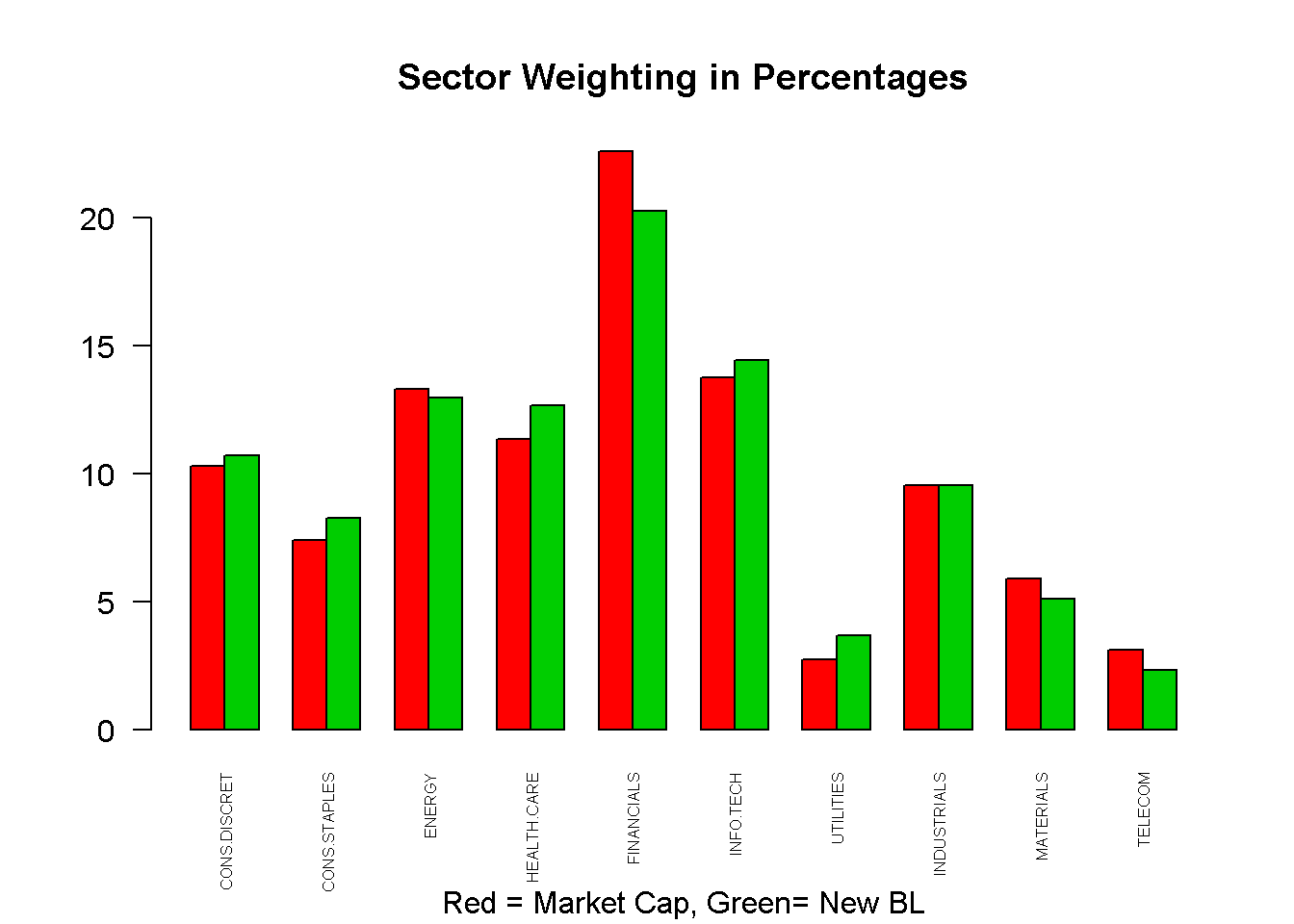### Calibrating Confidence Levels

Although we have just fledged out the model, we have yet to incorporate equity managers’ confidence levels. Confidence levels are on a percentage basis and help to eliminate the confusing fine-tuning of $$\tau$$ and $$\Omega$$. If we were 100% certain on our beliefs, the expected return equation is then

$E[R_{100\%}]=\pi+\tau\Sigma P^T(P\tau\Sigma P^T)^{-1}(Q-P\pi)$
$w^*_{100\%} = (\lambda\Sigma)^{-1}E[R_{100\%}]$

Define $$Tilt=(w_{100\%}-w_{mkt})*C$$ as a $$N\times 1$$ vector that specifies the degree of departure in sector weighting from 100% confidence level. Conceptually, if we were 100% in one belief, we would allocate based on $$w_{100\%}$$ which deviates from original optimal allocation ($$w_{mkt}$$) by a certain percentage. However, since we are only $$C\%$$ confident in the belief, we would only deviate by $$(w_{100\%}-w_{mkt})*C$$. Implementation is as follows (for each $$k$$):

1. Define Confidence Levels
2. Find $$E[R_{100\%}]$$ and $$w^*_{100\%}$$
3. Find $$Tilt$$
4. Find new confidence-incorporated weighting $$w^* = w_{mkt} + Tilt$$
5. Solve for $$\Omega_{k,k}$$ such that the squared difference between the confidence weighting and BL output weighting are minimized. BL outputing weighting is slightly different from the original formula such that it only contains the k-th view:

$\omega_k = [\lambda\Sigma]^{-1}[(\tau\Sigma)^{-1}+P_k^T\Omega_{k,k}^{-1}P_k]^{-1}][(\tau\Sigma)^{-1}\pi+P_k^T\Omega_{k,k}^{-1}Q_k]$

  conf.levels <- c(0.8,0.6,0.8,0.8,0.7,0.9,0.7) # Step 1
for(i in 1:K){
# Step 2
bl.er.100 <- pi + (tau*cov.mat%*%P[i,])*as.numeric((solve(P[i,]%*%(tau*cov.mat)%*%P[i,]))*(Q[i]-P[i,]%*%pi))
w.bl.100 <- solve(c(lambda)*cov.mat)%*%bl.er.100

#Step 3 & 4
tilt <- (w.bl.100-w.mkt)*conf.levels[i]
w.conf.k <- w.mkt + tilt

#Step 5
#define obj function
omega_solve <- function(omega, w.conf.k, lambda, sigma, tau, p, pi, q){
#omega <- omega/10000
w.single <- solve(c(lambda)*sigma) %*%
solve(solve(tau*sigma)+(1/omega*p)%*%t(p)) %*%
(solve(tau*sigma)%*%pi+c((1/omega)*p*q))
sum(((w.conf.k-w.single))^2)
}
#Solve
m <- seq(0.0000001,0.001,by=0.0000001)
d <- 1
e <- c()
for(j in m){
e[d] <- omega_solve(j,w.conf.k,lambda,cov.mat,tau,P[i,],pi,Q[i])
d <- d+1
}
omega[i,i] <- m[which.min(e)]
}

Let’s take a look at the final list of weights for SSIF sector allocation.

  bl.er <- solve(solve(tau*cov.mat)+t(P)%*%(solve(omega)%*%P)) %*% (solve(tau*cov.mat)%*%pi+t(P)%*%(solve(omega)%*%Q))
w.bl <- solve(c(lambda)*cov.mat)%*%bl.er
barplot(t(cbind(w.mkt*100,w.bl*100)), beside=TRUE, col=2:3, cex.names=0.5, las=2, main="Sector Weighting in Percentages", sub="Red = Market Cap, Green= New BL")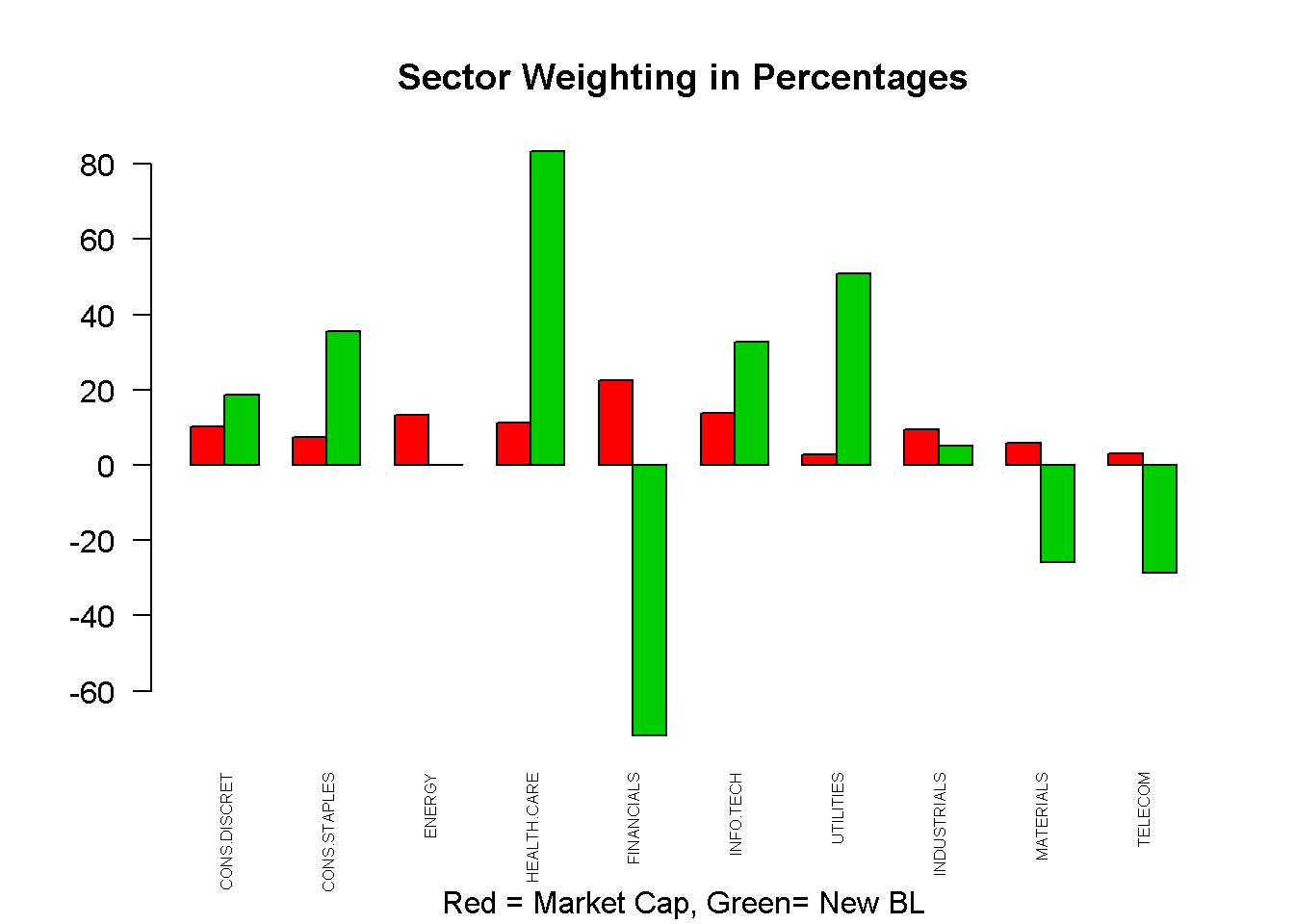Since we cannot short a sector, one simple workaround is to recalibrate $$\tau$$ until all weights are zero or more. The above chart is useful in seeing the exacerbated effect of the views vector.

  tau <- 0.000055 # This one happened to work well.
bl.er <- solve(solve(tau*cov.mat)+t(P)%*%(solve(omega)%*%P)) %*% (solve(tau*cov.mat)%*%pi+t(P)%*%(solve(omega)%*%Q))
w.bl <- solve(c(lambda)*cov.mat)%*%bl.er
barplot(t(cbind(w.mkt*100,w.bl*100)), beside=TRUE, col=2:3, cex.names=0.5, las=2, main="Sector Weighting in Percentages", sub="Red = Market Cap, Green= New BL")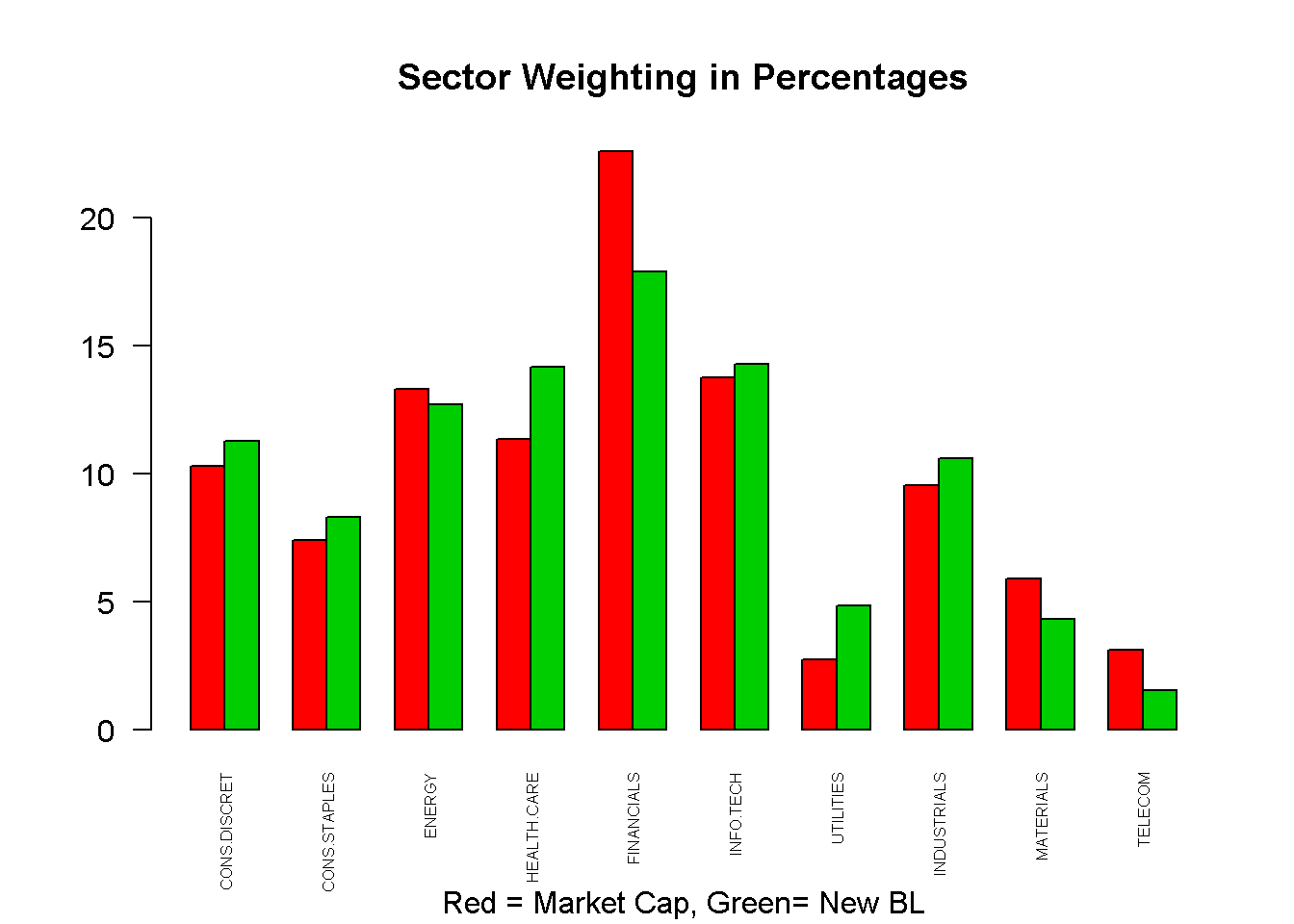### Conclusion and Final Subjective Adjustments

To summarize, in this report, I used the Black-Litterman model to estimate both sector returns and weighting. Taking equity managers’ beliefs on each sector, we create a new set of sector weightings that are consistent with managers’ beliefs both in terms of direction and confidence level.

Final subjective adjustments are made with the Portfolio Manager on sector weightings. They are as follows:

1. Since we do not currently own stocks in the Telecom sector, its weighting will be prorated equally to materials and Consumer Discretionary.
2. Financials is a sector we do not strongly feel confident in. The fact that the benchmark is heavily concentrated within financials calls for an additional downgrade. The effect matrix has been modified to adjust for this.
  # Final adjustments
w.bl[c(1,9)] <- w.bl[c(1,9)]+w.bl/2
w.bl <- 0

#Final plot
barplot(t(cbind(w.mkt.us*100,w.mkt.cn*100,w.bl*100)), beside=TRUE, col=c(6,7,3), cex.names=0.5, las=2, main="Final Sector Weighting in Percentages")
legend("topright", legend = c("US Benchmark W", "CN Benchmark W", "Optimal W"), col=c(6,7,3),pch=1,cex=0.8) # legend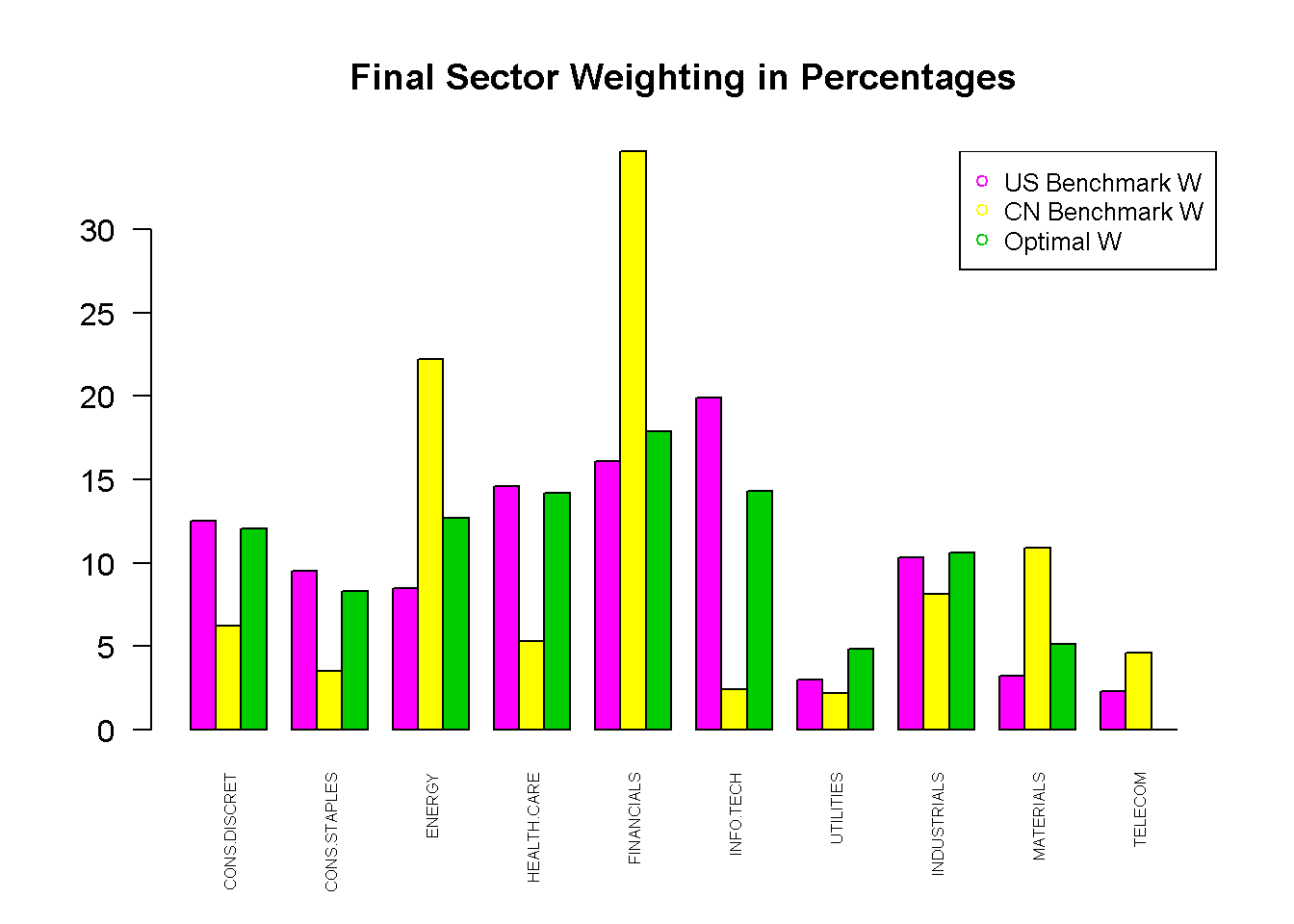The table below summarizes our final results:

Sectors Belief Confidence Level $$\Delta$$ in Weights
Consumer Discretionary Equal Weight 80% 1.76%
Consumer Staples Equal Weight 70% 0.91%
Energy Equal Weight -> Overweight 70% (0.60%)
Health Care Overweight 80% 2.82%
Financials Underweight - (4.7%)
Info. Technology Overweight 60% 0.53%
Utilities Overweight 80% 2.10%
Industrials Equal Weight 90% 1.08%
Materials Underweight - (0.80%)
Telecom Underweight - (3.11%)